#Quality Digest

Featured Product
This Week in Quality Digest Live
Statistics Features
Donald J. Wheeler
What do predictable processes have in common with chaos?
William A. Levinson
People can draw the wrong conclusions due to survivor, survey, and bad news bias.
Donald J. Wheeler
Does your approach do what you need?
Paul Laughlin
Correlation vs. causality
Donald J. Wheeler
In spite of what everyone says to the contrary
Statistics News
New capability delivers deeper productivity insights to help manufacturers meet labor challenges
Day and a half workshop to learn, retain, and transfer GD&T knowledge across an organization
Elsmar Cove is a leading forum for quality and standards compliance
InfinityQS’ quality solutions have helped cold food and beverage manufacturers around the world optimize quality and safety
User friendly graphical user interface makes the R-based statistical engine easily accessible to anyone
Collect measurements, visual defect information, simple Go/No-Go situations from any online device
Good quality is adding an average of 11 percent to organizations’ revenue growth
Ability to subscribe with single-user minimum, floating license, and no long-term commitment
A guide for practitioners and managers
Statistics

## When Are Instruments Equivalent? Part 2

### Do the instruments have the same amount of measurement error?

Published: Monday, May 6, 2019 - 12:03Donald J. WheelerJames Beagle III

All articles in this series:

Last month we provided an operational definition of when measurement systems are equivalent in terms of bias. Here we will look at comparing the within-instrument measurement error between two or more systems.

Once again we must emphasize that it makes no sense to seek to compare measurement systems that do not display a reasonable degree of consistency. Consistency must be demonstrated, it cannot be assumed, and a consistency chart is the simplest way to do this.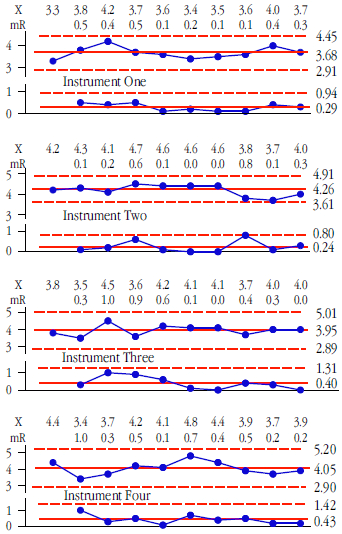Figure 1: Consistency charts for instruments 1, 2, 3, & 4

So once more we begin with consistency charts. Figure 1 shows the data and consistency charts for instrument Nos. 1, 2, 3, and 4. Figure 2 shows the data and consistency charts for instrument Nos. 5, 6, 7, and 8. Each of these eight instruments was used to measure the same standard 10 times. None of these charts show any evidence of inconsistency. But the question of whether these eight instruments are equivalent remains unanswered. Here we shall begin with the question of whether they all show equivalent amounts of measurement error.Figure 2:  Consistency charts for instruments 5, 6, 7, & 8

### Checking for equivalency of measurement error

The moving ranges in figures 1 and 2 represent measurement error. The average moving ranges for these eight instruments are, respectively, 0.289, 0.244, 0.400, 0.433, 0.322, 0.411, 0.444, and 0.833. To test if these average moving ranges are equivalent we shall use the Analysis of Mean Moving Ranges, ANOMmR (pronounced a-nom-m-r).

The ANOMmR chart compares m average moving ranges where each average moving range is based upon (k–1) two-point moving ranges. That is, each average moving range comes from an XmR chart that has a baseline of k original data.

The central line of an ANOMmR chart is the grand average of the m average moving ranges. For the eight XmR charts in figures 1 and 2 the grand average moving range is 0.4222.

The upper and lower detection limits of an ANOMmR chart are found by multiplying the grand average moving range by scaling factors. These ANOMmR scaling factors will depend upon your choice for the risk of a false alarm (the alpha level), the number of average moving ranges being compared (denoted here by m), and the number of original X values in each of the XmR charts (denoted here by k). Tables of these scaling factors are given at the end of this article.

For this example we are comparing the average moving ranges from m = 8 different XmR charts, each of which is based upon k = 10 original values. We choose to use an alpha-level of 5 percent because this is the traditional, default alpha level for a one-time analysis. From the tables we find our scaling factor for the upper ANOMmR detection limit is UL = 1.871, and the scaling factor for the lower ANOMmR detection limit is LL = 0.375. With our grand average moving range value of 0.4222 we find: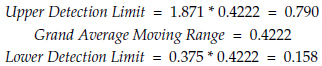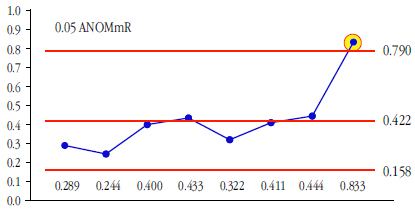Figure 3: 5% analysis of mean moving ranges

Figure 3 shows that instrument No. 8 has a detectably different amount of measurement error than the other seven instruments. (This was not immediately apparent in figure 2 simply because, like charts produced by most software, all of these charts were scaled so that the graph fit into a fixed size format, rather than using a fixed scale for all the graphs. Thus, the chart for instrument No. 8 with limits 4.4 units apart [1.8 to 6.2] is shown the same size as the chart for instrument No. 2 with limits only 1.5 units apart [3.6 to 4.9]).

Now that we know instrument No. 8 has a different amount of measurement error we must characterize it separately from the others. Instrument No. 8 has an average of 4.01 units, and an average moving range of 0.8333 units. Dividing the average moving range by d2 = 1.128 gives an estimate of SD(E) of 0.74 units, and multiplying this by 0.675 results in a probable error of 0.50 units. So while instrument No. 8 records values to a tenth of a unit, the values are only good to about one-half unit. Half the time they will err by one-half unit or more, and half the time they will err by one-half unit or less.

In contrast to what we see with instrument No. 8, the remaining seven instruments appear to have equivalent average moving ranges. So, we can combine these seven values to obtain a new grand average moving range of 0.3635 units. This translates into a common estimate of measurement error for the remaining seven instruments of SD(E) = 0.3222 units, and a common probable error of 0.22 units. Thus, instruments No. 1 through No. 7 give values with a precision that will err by less than one-quarter unit at least half the time.

So, by using ANOMmR we can separate these eight instruments into seven that have equivalent amounts of measurement error and one that has roughly twice as much measurement error.

### Checking for bias between instruments with ANOM

To check for bias effects between the seven instruments having equivalent amounts of measurement error we proceed as described last month in part one. The XmR charts of figures 1 and 2 show that these measurement systems are consistent, and this consistency justifies reorganizing the data from the seven XmR charts into k = 7 subgroups of size n = 10 and using ANOM to check for detectable biases between instruments. (Instrument No. 8 was not included in this analysis because we already know it is in a league of its own.) See figure 4 for this reorganization of the data. (While k denoted the number of original values in an XmR chart above, here the symbol k is used to represent the number of subgroups. The authors apologize for using the symbol k for two different things, but this is all part of the standard notation for these techniques.)Figure 4: Seven subgroups of size 10

For the seven instruments shown in figure 4, the grand average is 4.029, and the average of the seven subgroup ranges is 1.014 units.

According to the formulas given in figure 7 of Part One, an unbiased estimate of the standard deviation of the k = 7 subgroup averages in figure4 is: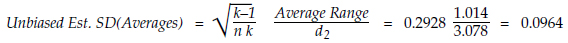And this estimate has approximately 55 degrees of freedom.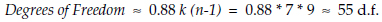ANOM detection limits have the form: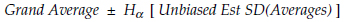Where the ANOM scaling factor H may be found in the tables in Part One. We shall use the traditional alpha level for one-time analyses of 5 percent. We are comparing k = 7 averages, and our estimate of dispersion has about 55 degrees of freedom. Rounding this down to the tabled value of 40 degrees of freedom, with k = 7 and alpha = 0.05, we find our ANOM scaling factor to be 2.791. So our ANOM detection limits are:Figure 5: ANOM for instrument bias effects

Here we find instruments No. 1 and No. 5 with a detectable bias while instrument No. 2 comes close to being detectably different from the grand average of all seven instruments.

### Recentering the limits

Figure 5 compares each subgroup average with the grand average. But when detectable differences are present the grand average is the average of unlike things. This makes the grand average a rather arbitrary point for comparison. A more meaningful reference point in this case would be the average of instruments No. 3, No. 4, No. 6, and No. 7, which is 3.895. If we shift the ANOM limits to be centered on 3.895 we get: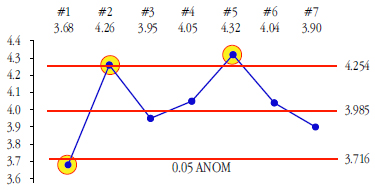Figure 6: ANOM for instrument bias effects with shifted central line

Now we can logically separate our eight instruments into four groups. The first group consists of instruments No. 3, No. 4, No. 6, and No. 7 which are fully equivalent to each other: Figure 6 shows that they have no detectable bias relative to each other and figure 3 shows that they all have equivalent amounts of measurement error.

The second group consists of instruments No. 2 and No. 5. These two instruments are biased relative to the first group even though they have the same amount of measurement error. Instrument No. 2 has a relative bias of 0.275 units compared to the first group, while instrument No. 5 has a relative bias of 0.335 units. In part one we learned that bias effects smaller than 1.128 SD(E) are too small to make a difference in practice. Here the common estimate of SD(E) is 0.3222 units, so biases smaller than 0.36 units are negligible in practice. If we only had the first and second groups of instruments, they could be used together with little impact upon the quality of the readings.

While recalibration to remove detectable biases is desirable, recalibration can become difficult as the bias gets smaller than the measurement error. However, artificial adjustments of the readings are still possible. The readings from instruments No. 2 and No. 5 could be adjusted down by 0.3 units if complete parity with group one is desirable.

The third group consists of instrument No. 1 alone. While it has the same amount of measurement error as the instruments in the first two groups, it, is biased by –0.305 units relative to the first group. Once again recalibration is desirable, but may prove difficult. If recalibration is not feasible, and complete parity is desired between instrument No. 1 and the first two groups, we could adjust readings from instrument No. 1 by simply adding 0.3 units.

Instrument No. 1 is biased relative to instrument No. 2 by 0.58 units or 1.80 SD(E), and it is biased relative to instrument No. 5 by 0.64 units or 1.98 SD(E). These biases are large enough that it is impractical to treat the raw readings from instrument No. 1 as equivalent in practice to raw readings from Instrument No. 2 or No. 5. However, if it is reasonable to adjust the readings from instruments No. 1, No. 2, and No. 5 as described above, all seven instruments in figure 6 will produce values that can be considered to be fully equivalent in practice.

The fourth group consists of instrument No. 8 which has twice the measurement error of the other seven instruments. With an average of 4.01, Instrument No. 8 would fall well within the limits in figure 6, so we conclude that instrument No. 8 shows no real bias relative to the first group.

### Bias relative to a known standard

All bias is relative. We have already identified the biases of these seven instruments relative to each other, and have identified the appropriate adjustments to be made to the readings for three instruments. Since this study was carried out with a known standard that had an accepted value of 4.00, we can also talk about the bias of these seven instruments relative to the master measurement method represented by the known standard. The simplest way to do this is to re-center the ANOM at the accepted value for the known standard. Thus, our limits for instrument Nos. 1 through 7 become:When we include the adjustments suggested earlier for instruments No. 1, No. 2, and No. 5, we end up with figure 7.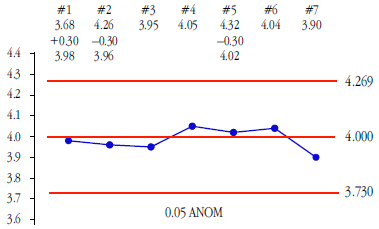Figure 7:  ANOM centered on accepted value of known standard

Thus, these seven instruments can all be made to operate without any detectable bias relative to the master measurement method represented by the known standard.

So, by using ANOMmR and ANOM we have characterized our eight instruments, we know how to make instrument Nos. 1 through 7 equivalent, and we know that instrument No. 8 has twice as much measurement error as the other seven. We know how these instruments work relative to the master measurement method, and we have simple graphs to use in communicating these findings to others.

### ANOMmR for the example from Part One

In Part One we had three instruments, A, B, and C. By informally comparing the average moving ranges it was said that these three instruments appeared to have equivalent amounts of measurement error. Here we shall use ANOMmR to examine this idea. The consistency charts for these three instruments had k = 30 data each, and the average moving ranges were, respectively, 4.17 units, 3.50 units, and 3.93 units.

The grand average moving range is 3.867. With k = 30, and m = 3, and with a traditional alpha level of 5 percent, we find ANOMmR scaling factors of LL = 0.685 and UL = 1.337.Figure 8: ANOMmR for the three instruments in Part One

Since all three average moving ranges fall within these limits we find that there is no detectable difference in measurement error between these three instruments.

### Summary

When comparing m different situations using XmR charts for each situation we often want to know if the different situations all have the same amount of variation. The ANOMmR approach given here allows an easy way to answer this question and communicate the result to others using a simple, understandable graph.

In Part Three we shall look at comparing instruments using multiple standards.

### About the tables for ANOMmR

The following tables define scaling factors to use when comparing m average moving ranges, each of which comes from an XmR chart for k individual values. Here each average moving range will be based on (k–1) two-point moving ranges, and the average of the m average moving ranges will be the grand average moving range. The tabled scaling factors were found by simulation studies starting with 20 million observations from a standard normal distribution. These values were partitioned into sets of k values and the (k–1) moving ranges were found. Next the average moving range for each set of k values was found.

For a given value of k, these average moving ranges were then organized into groups of size m, and for each group the minimum and the maximum were each divided by the average for that group. When these two ratios were computed for 10,000 groups for a given combination of m and k, each of the ratios were then organized into a histogram and the appropriate percentiles were found. For the ratios of minimum average moving range to grand average moving range the 0.005, 0.025, and 0.050 percentiles were found and used to define the LL scaling factors. For the ratios of maximum average moving range to grand average moving range the 0.950, 0.975, and 0.995 percentiles were found and used to define the UL scaling factors. Finally, the percentiles from two or more such histograms were averaged together to get the values given in the table. Based on the convergence of these percentiles the 2,016 tabled values are, by and large, good to three decimal places.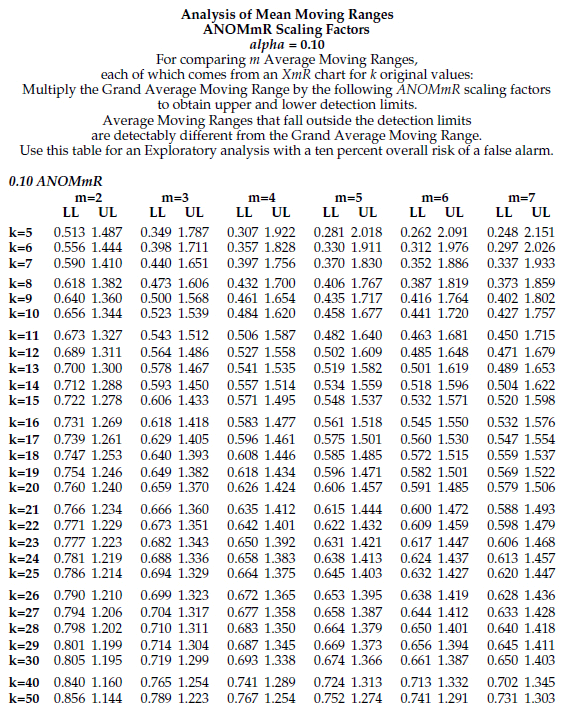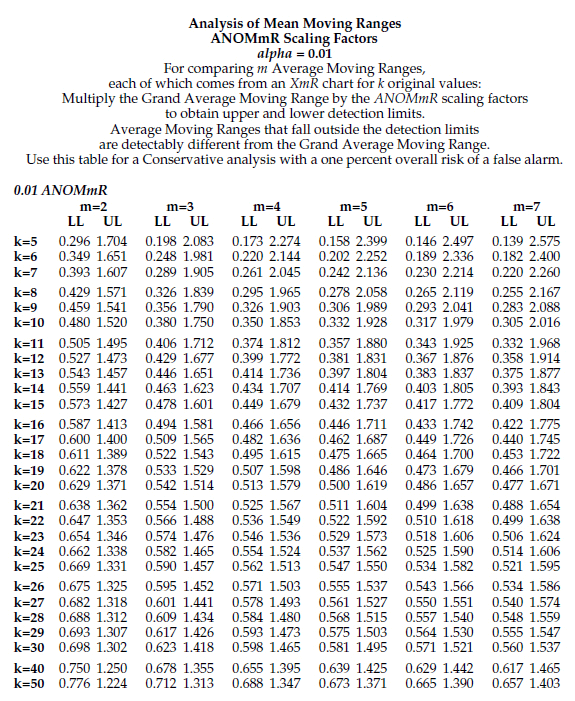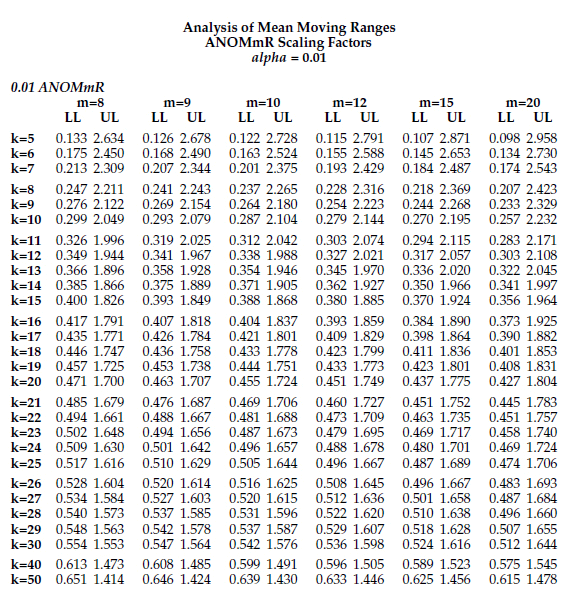### Discuss### Donald J. Wheeler

Find out about Dr. Wheeler’s virtual seminars for 2022 at www.spcpress.com. Dr. Wheeler is a fellow of both the American Statistical Association and the American Society for Quality who has taught more than 1,000 seminars in 17 countries on six continents. He welcomes your questions; you can contact him at djwheeler@spcpress.com.### James Beagle III

James Beagle III is a lapsed CQE, CRE, and C6SBB (asking the wrong questions and obsessing about impractical concepts, before realizing what is important) with 35+years experience in process, quality, and reliability engineering. He primarily works with a large manufacturing base supplying data storage component products, where he develops tools for data analysis, designs experiments for product improvements, and works on product and process qualification. In his free time he works on numerical modeling (and is also known to have guitars at home). He can be reached at james@idealstates.net.